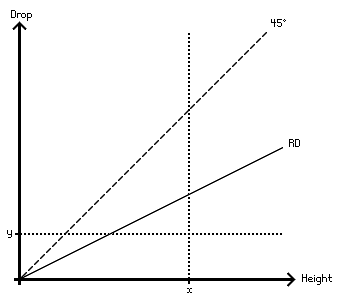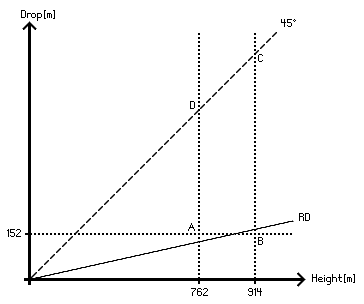www.simonsmountainlists.com/bagging/relativedrop

Relative drop

.

#### The drawback of drop

Drop (or relative height, or prominence) is now a widely-used concept. It's a simple way to quantify the 'sticking-upness' of a peak - so simple, you just have to count it. However, it applies the same measure to a 200m lump as it does to a 1200m mountain. The logical progression is therefore to divide the drop by the height, to get drop as a proportion of height.

#### A new measure!

I called this concept 'relative relative height', or more simply relative drop when I first used it in a list in March 2002. It means that a 1000m mountain with 200m of drop has a relative drop of 20%. A 600m mountain would be equivalent in relative drop terms if it had 120m of drop.

#### A graph

Figure 1 shows relative drop alongside the two most commonly used measures: height and drop. Height is on one axis and drop is on the other. All peaks have a certain height and a certain drop and can therefore be plotted on this graph. Along the 45 degree line, height equals drop. All peaks which have no higher peak reachable except by dropping to sea level - all island high-points - will be on this line. It's impossible (in the absence of quarrying) for a peak's drop to be greater than its height, so no peaks will be to the left of the 45 degree line. A typical list will stipulate a minimum height (x) and a minimum drop (y) and will therefore be the set of all peaks both to the right of x and above y.

The relative drop (RD) line links points with the same drop-to-height ratio. All points along the RD line have the same RD value, calculated as drop divided by height.

Figure 1#### Suitable levels of relative drop

It might be that a relative drop of 15% works well for some ranges. This is the relative drop of a 1000m Munro with 150m of drop. However, we can be reasonably precise... as follows.

Take the Corbetts as an example. Corbetts must be at least 2500ft (762m) high but no more than 2999ft (914m) high, and have at least 500ft (152m) of drop. What's a suitable level of relative drop for this group of mountains? First let's set upper and lower limits on what our relative drop figure can reasonably be, where reasonableness means sticking to the spirit of the Corbett criteria above.

In the following, a Theoretical Corbett is a mountain that does not necessarily exist but that would meet the Corbett criteria if it did; the Floor is the minimum height of a mountain in the group (762m for the Corbetts); and the Ceiling is the maximum height of a mountain in the group (914m for the Corbetts). Figure 2 shows the area ABCD in which Theoretical Corbetts exist, which we can call the Corbett zone.

Figure 2(i) For the relative drop (RD) criterion to include all Theoretical Corbetts, it would need to be:

 RD <= Drop / Ceiling = 152 / 914 = 16% (1)

(The RD line would pass though point B.)

(ii) For the relative drop criterion (RD) to exclude all Theoretical Corbetts apart from those with RDs at least as great as the RD of the Theoretical Corbett with the lowest possible height and the lowest possible drop, it would need to be:

 RD >= Drop / Floor = 152 / 762 = 20% (2)

(The RD line would pass though point A.)

The ideal relative drop for the Corbetts will therefore be between 16% and 20% inclusive.

However, we can arrive at a precise figure if we stipulate that the area in the Corbett zone in Figure 2 above must be the same under the relative drop regime as under the drop regime. The result will be 18%, the average of 16% and 20%, because the height at which the relative drop line must cross the drop=152m line must be the average of the floor and the ceiling for there to be as much area lost as gained.

So how about a modification to the Corbetts list that replaces the 152m drop criterion with an 18% relative drop criterion? First, what would this 18% level mean for the list's minimum drop levels at the floor and at the ceiling? To find drops just rearrange the RD equation above. At the floor the minimum drop would be:

 Drop = Floor x RD = 762m x 18% = 138m (3)

At the ceiling the minimum drop level would be:

 Drop = Ceiling x RD = 914m x 18% = 166m (4)

So in a Corbett list including only those peaks with relative drops of at least 18%, a mountain 762m high would need only 138m of drop, but a mountain 914m high would need 166m of drop. Purists might object to debasing the Cotbett list to allow in any mountain with less than 152m of drop, in which case there are two options:

(i) We could require 20% relative drop, where 20% is the figure in equation 2 above that excludes all except those with RDs at least as great as the RD of the Theoretical Corbett with the lowest possible height and the lowest possible drop; or

(ii) The list could use the criterion '18% relative drop and 152m drop', effectively bringing in the relative drop measure only for the higher mountains. The height above which the RD criterion kicks in instead of the drop criterion is just the average of the floor and the ceiling: 844m.

#### Uniform distribution assumption

This result of course assumes that Corbetts are distributed uniformly throughout the Corbett zone. This is unlikely to be the case. Island high-points will sit on the 45 degree line, while the bigger number of non-island-high-points will congregate towards the lower drop levels. It is likely that a relative drop line fitted in order to give the same number of Corbetts under both regimes (not in order to give the same area in the zone) will therefore have a shallower gradient (lower relative drop).

#### Should we use drop or drop2 to find relative height?

Clearly it matters which definition of drop we use. To recap, drop is the standard measure: the difference between a peak's height and the height of the highest col connecting that peak to a higher peak. Drop 2 is the difference between a peak's height and the height of the highest col connecting it to any other peak. Using drop to calculate relative drop would mean Snowdon had a relative drop of 1000m/1085m = 92.2%. Using drop2, the relative drop is 95m/1085m = 8.8%. The difference is (of course) what we use as the base from which Snowdon is rising up. Either it's the shallow col with Carnedd Ugain (drop2) or it's a point somewhere on the way to the Highlands, where the only higher peaks in mainland Britain are to be found (drop). If we use the drop2 method, then we end up with a reasonably neat spread of relative drops. For instance, using drop2 the fifteen Welsh 3000s have relative drops of between 4.7% and 25.0%. However, a better reason for using drop2 to find relative drop is that it fits in with the rationale for using relative drop in the first place, which is to quantify the sticking-upness of a hill relative to its neighbours. This makes sense given that the info is most useful to us when we're in the group and climbing the peaks.

In the Welsh 3000s list below we can see the effects of using both types of drop to find relative drop.

 No. Peak Massif Ht[m] Drop[m] Drop2[m] Relative drop[%] Relative drop2[%] 123456789101112131415 Snowdon Carnedd Ugain Carnedd Llewelyn Carnedd Dafydd Glyder Fawr Glyder Fach Pen yr Ole Wen Foel Grach Yr Elen Y Garn Foel Fras Garnedd Uchaf Elidir Fawr Crib Goch Tryfan Snowdon Snowdon Carneddau Carneddau Glyderau Glyderau Carneddau Carneddau Carneddau Glyderau Carneddau Carneddau Glyderau Snowdon Glyderau 1085 1065 1064 1044 999 994 978 976 962 947 942 926 924 923 915 999 75 752 114 640 74 48 46 62 237 62 46 224 73 195 95 75 134 114 79 74 48 46 62 237 62 46 224 73 195 92.2 7.0 70.7 10.9 64.1 7.4 4.9 4.7 6.4 25.0 6.6 5.0 24.2 7.9 21.3 8.8 7.0 12.6 10.9 7.9 7.4 4.9 4.7 6.4 25.0 6.6 5.0 24.2 7.9 21.3